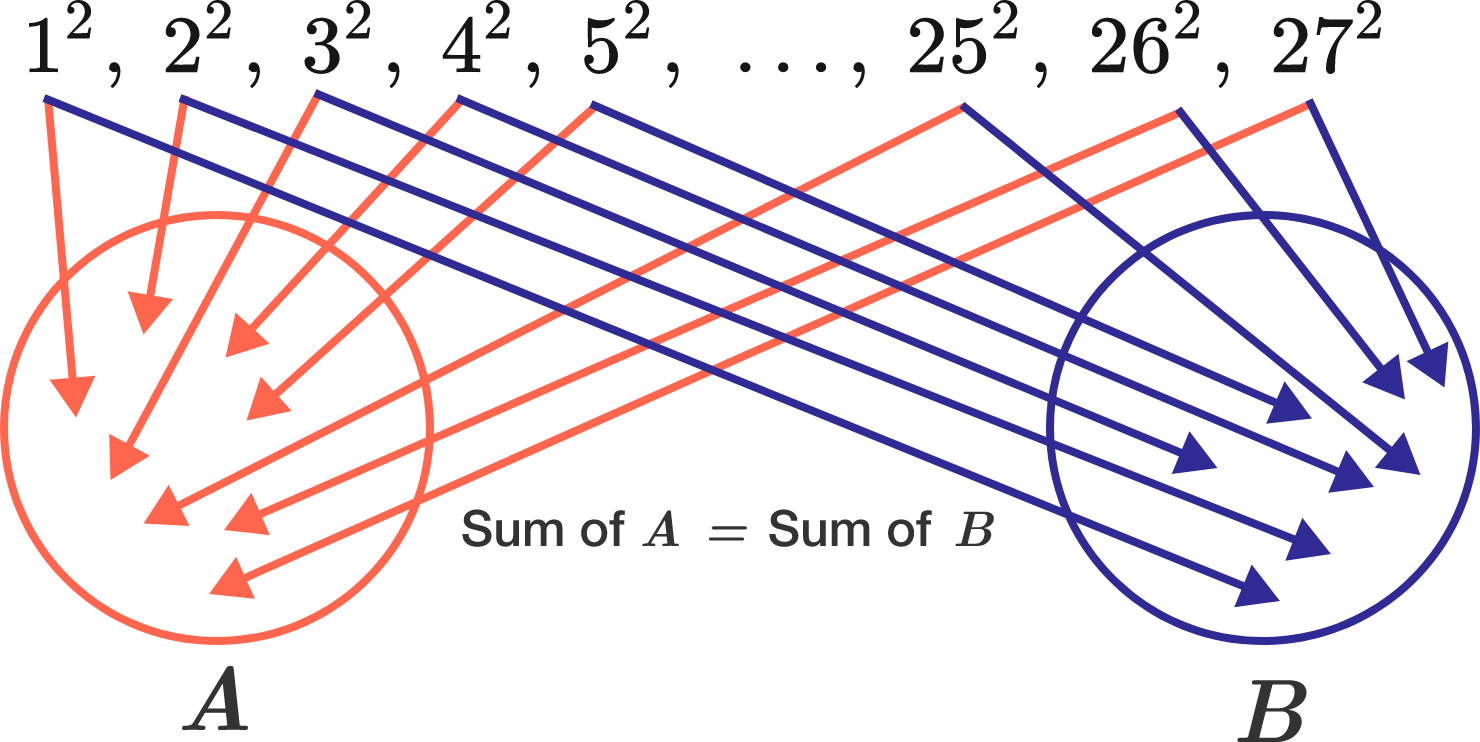# Regions

Is it possible to place every one of the following numbers $1^2,\ 2^2,\ 3^2,\ 4^2,\ 5^2,\ \dots,\ 25^2,\ 26^2,\ 27^2$ into either of the two regions $A$ and $B$ below such that the sums of the numbers in each region are equal?×

Problem Loading...

Note Loading...

Set Loading...